Refer to our Texas Go Math Grade 3 Answer Key Pdf to score good marks in the exams. Test yourself by practicing the problems from Texas Go Math Grade 3 Lesson 4.5 Answer Key Use Place Value to Add.

Unlock the Problem

Dante is planning a trip to Illinois. His airplane leaves from Dallas, Texas and stops in Tulsa, Oklahoma. Then it flies from Tulsa to Chicago, Illinois. How many miles does Dante fly?Estimate: 236 + 585 = _____________

STEP 1:
Add the ones. Regroup the ones as tens and ones.STEP 2:
Add the tens. Regroup the tens as hundreds and tens.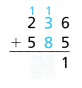STEP 3: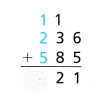236 + 585 = _________
So, Dante flies _________ miles.
Since _________ is close to the estimate of _________ the answer is reasonable.

Remember to add the regrouped ten and hundred.

You can also use the Commutative Property of Addition to check your work. Change the order of the addends and find the sum.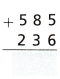821 miles
Commutative property
X+Y = Y+X
585 + 236 = 236 + 585 =  821
585 is rounded to 600 and 236 is rounded to 200
600 + 200 = 800 estimated
Explanation:
236 + 585 = 821
So, Dante flies 821 miles.
Since 821 is close to the estimate of 800 the answer is reasonable.

Example

Add. 173 + 102 + 328
Estimate. 200 + 100 + 300 = _________

STEP 1:
Add the ones. Regroup the ones as tens and ones.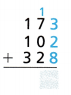STEP 2:
Add the tens. Regroup the tens as hundreds and tens.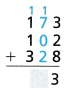STEP 3:So, 173 + 102 + 328 = ____________.
Explanation:
100  +  200  + 300 = 600
173 + 102 + 328 = 603
(173 + 102) + 328 = 173 + (102 + 328)     (Associative property (x+y)+z = x+(y+z) )
175 + 328 = 173 +430 = 603
Since 603 is close to the estimate of 600 the answer is reasonable.

Math Talk
Mathematical Processes

Explain how you can compute 403 + 201 mentally.
Explanation:
403 is rounded to 400 and 201 is rounded to 200 as they are nearer to hundred
400 + 200 =600

Share and Show

Estimate. Then find the sum.

Question 1.
Estimate: ____________251 + 345 = 596
300 + 300 = 600
Since 596 is close to the estimate of 600 the answer is reasonable.
Explanation:
251 is above 250, so 251 is rounded to 300 and
345 is below 350, so 345 is rounded to 300
the sum of estimated numbers
300 + 300 = 600

Question 2.
Estimate: _____________
276 + 410 + 314 = ___________

300 + 400 + 300 = 1000
Explanation:
276 is rounded to 300
410 is rounded to 400
314 is rounded to 300
300 + 400 + 300 = 1000
Since 1000 is same to the estimate of 1000 the answer is equal and reasonable.

Problem Solving

Practice: Copy and Solve Estimate. Then solve.

Question 3.
253 + 376
253 + 376 = 629
Explanation:
253 is nearer to 250, so 253 is rounded to 250 and
376 is nearer to 380, so 376 is rounded to 380
the sum of estimated numbers
250 + 380 = 620
Since 629 is close to the estimate of 620 the answer is reasonable.

Question 4.
654 + 263
654 + 263 = 917
Explanation:
654 is nearer to 655, so 654 is rounded to 655 and
263 is nearer to 265, so 263 is rounded to 265
the sum of estimated numbers
265 + 655  = 920
Since 917 is close to the estimate of 920 the answer is reasonable.

Question 5.
321 + 439
321 + 439 = 760
Explanation:
321 is rounded to 320 and 439 is rounded to 440
the sum 320 + 440 = 760

Question 6.
482 + 323
482 + 323 = 805
Explanation:
482 is rounded to 500 and 323 is rounded to 300
the sum 500 + 300 = 800

Question 7.
182 + 321
Explanation:
182 is rounded to 200 and 321 is rounded to 300
the sum 200 + 300 = 500
Since 503 is close to the estimate of 500 the answer is reasonable.

Question 8.
701 + 108
Explanation:
701 is rounded to 700 and 108 is rounded to 100
the sum 700 + 100 = 800
Since 809 is close to the estimate of 800 the answer is reasonable.

Question 9.
543 + 372
543 + 372 = 915
Explanation:
543 is rounded to 500 and 372 is rounded to 400
the sum 500 + 400 = 900
Since 915 is close to the estimate of 900 the answer is reasonable.

Question 10.
516 + 326
516 + 326 = 842
Explanation:
516 is rounded to 500 and 326 is rounded to 300
the sum 500 + 300 = 800
Since 842 is close to the estimate of 800 the answer is reasonable.

H.O.T. Algebra Find the unknown digits.

Question 11.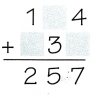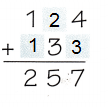Explanation:
When two numbers are added, the sum is the same regardless of the order of the addends .
Commutative property
124 + 133 = 133 + 124 = 257

Question 12.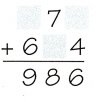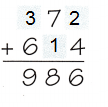Explanation:
When two numbers are added, the sum is the same regardless of the order of the addends .
Commutative property
372 + 614 = 614 + 372 = 986

Question 13.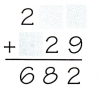Explanation:
When two numbers are added, the sum is the same regardless of the order of the addends .
Commutative property
253 + 429 = 429 + 253 = 682

Question 14.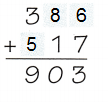Explanation:
When two numbers are added, the sum is the same regardless of the order of the addends .
Commutative property
386 + 517 = 517 + 386 = 903

Unlock the Problem

Question 15.
H.O.T. Multi-Step A plane flew 187 miles from New York City New York to Boston, Massachusetts. it then flew 273 miles from Boston to Philadelphia, Pennsylvania. The plane flew the same distance on the return trip. How many miles did the plane fly?(A) 460 miles
(B) 820 miles
(C) 900 miles
(D) 920 miles
Option (D)
Explanation:
A plane flew 187 miles from New York City New York to Boston, Massachusetts,
it then flew 273 miles from Boston to Philadelphia, Pennsylvania.
Total miles the plane fly
187 + 273 + 187 + 273 = 920

a. What do you need to find?
Total distance a plane flew
b. What is an estimate of the total distance?
900 miles
Explanation:
185 + 275 + 185 + 275 = 920
187 + 273 + 187 + 273 = 920

c. Show the steps you used to solve the problem.
187 is rounded to 185 and 273 is rounded to 275 for estimate
the total is 185 + 275 + 185 + 275 = 920

d. Complete the sentences.
The plane flew _________ miles from New York City to Boston.

Then it flew _________ miles from Boston to Philadelphia.

It flew _________ miles from New York City to Boston to Philadelphia.

The total distance is ________ miles round trip.
The plane flew 187 miles from New York City to Boston.

Then it flew 273 miles from Boston to Philadelphia.

It flew  460 miles from New York City to Boston to Philadelphia.

The total distance is 920  miles round trip.

e. Fill in the bubble for the correct answer choice above.
Explanation:
A plane flew 187 miles from New York City New York to Boston, Massachusetts,
it then flew 273 miles from Boston to Philadelphia, Pennsylvania.
Total miles the plane flew
187 + 273 + 187 + 273 = 920

Question 16.
Evaluate The Colorado River is 119 miles longer than the Kansas River. The Kansas River is 743 miles long. How long is the Colorado River?
862 miles
Explanation:
The Colorado River is 119 miles longer than the Kansas River.
The Kansas River is 743 miles long.
119 +743 = 862

Question 17.
Multi-Step Mr. Miller drove 568 miles last week. This week he drove 308 miles on Tuesday and 97 miles on Wednesday. What was the number of miles he drove in these two weeks?
973 miles
Explanation:
Mr. Miller drove 568 miles last week.
This week he drove 308 miles on Tuesday and 97 miles on Wednesday.
the number of miles he drove in these two weeks
568 + (308 + 97)
568 + 405 = 973

Fill in the bubble for the correct answer choice. Use place value to solve.

Question 18.
Joshua and Daniel’s hot air balloon rises 218 feet. Then it rises 124 feet more. How many feet did the hot air balloon rise in all?
(A) 332 feet
(B) 334 feet
(C) 342 feet
(D) 432 feet
Option (C)
Explanation:
Joshua and Daniel’s hot air balloon rises 218 feet.
Then it rises 124 feet more.
Total feet the hot air balloon rise in all
218 + 124 = 342

Question 19.
Emily watched two movies. The first movie was 125 minutes long. The second movie was 112 minutes long. How many minutes did Emily spend watching the two movies?
(A) 230 minutes
(B) 237 minutes
(C) 257 minutes
(D) 335 minutes
Option (B)
Explanation:
The first movie was 125 minutes long.
The second movie was 112 minutes long.
Total minutes Emily spent watching the two movie
125 + 112 = 237

Question 20.
Multi-Step Ava saved 245 pennies in April. She saved 365 pennies in May and saved 132 pennies June. How many pennies did Ava save in all?
(A) 610
(B) 632
(C) 700
(D) 742
Option (D)
Explanation:
Ava saved 245 pennies in April.
She saved 365 pennies in May and
saved 132 pennies June.
Total pennies Ava saved in all
245 +365 + 132 =742

Texas Test Prep

Question 21.
The Willard family collected cans for recycling. They collected 451 cans on Thursday, 246 cans on Friday, and 222 cans on Saturday. How many cans did the Willards collect for recycling on those three days?
(A) 819
(B) 919
(C) 468
(D) 697
Explanation:
They collected 451 cans on Thursday,
246 cans on Friday,
and 222 cans on Saturday.
Total cans the Willards collect for recycling on those three days
451 + 246 + 222 = 919

### Texas Go Math Grade 3 Lesson 4.5 Homework and Practice Answer Key

Estimate. Then find the sum.

Question 1.
Estimate: __________Estimate: 1000
Explanation:
Add the ones. Regroup the ones as tens and ones.
Add the tens. Regroup the tens as hundreds and tens.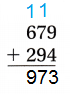Question 2.Estimate: 800
Explanation:
Explanation:
Add the ones. Regroup the ones as tens and ones.
Add the tens. Regroup the tens as hundreds and tens.Question 3.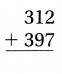Estimate: 700
Explanation:
Explanation:
Add the ones. Regroup the ones as tens and ones.
Add the tens. Regroup the tens as hundreds and tens.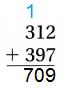Question 4.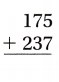Estimate: 400
Explanation:
Explanation:
Add the ones. Regroup the ones as tens and ones.
Add the tens. Regroup the tens as hundreds and tens.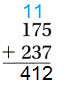Question 5.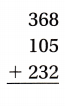Estimate: 700
Explanation:
Explanation:
Add the ones. Regroup the ones as tens and ones.
Add the tens. Regroup the tens as hundreds and tens.Question 6.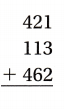Estimate: 1000
Explanation:
Explanation:
Add the ones. Regroup the ones as tens and ones.
Add the tens. Regroup the tens as hundreds and tens.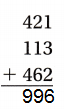Problem Solving

Question 7.
Mr. Conti drove 132 miles on Highway 9. He then drove 179 miles on Highway 16. How many miles did he drive altogether?
318 miles
Explanation:
Mr. Conti drove 132 miles on Highway 9.
He then drove 179 miles on Highway 16.
Total miles he drove altogether
139 + 179 = 318 miles

Question 8.
A bus takes a route from New York City to Washington, D.C. that is 226 miles.- How many miles is the round trip?
452 miles
Explanation:
A bus takes a route from New York City to Washington, D.C. that is 226 miles.
Total number of miles round the trip
226 + 226 = 452

Lesson Check

Question 9.
A bookstore sold 453 books on Saturday and 369 books on Sunday. How many books were sold on both days?
(A) 812
(B) 822
(C) 722
(D) 712
Option (B)
Explanation:
A bookstore sold 453 books on Saturday and 369 books on Sunday.
Total books sold on both days
453 + 369 = 822

Question 10.
Sandra drove 121 miles from San Diego to Los Angeles. The next day she drove 382 miles from Los Angeles to San Francisco. How many miles did she drive?
(A) 403 miles
(B) 502 miles
(C) 513 miles
(D) 503 miles
Option (D)
Explanation:
Sandra drove 121 miles from San Diego to Los Angeles.
The next day she drove 382 miles from Los Angeles to San Francisco.
Total miles she drove
121 + 382 = 503

Question 11.
A theater has 348 seats on the bottom level and 376 seats in the balcony. How many seats are there?
(A) 724
(B) 624
(C) 714
(D) 614
Option (A)
Explanation:
A theater has 348 seats on the bottom level and 376 seats in the balcony
Total seats in balcony
348 + 376 = 724

Question 12.
Julie had to take two different planes on her trip. The first plane had 234 passengers. The second plane had 167 passengers. How many passengers were on both flights?
(A) 411
(B) 301
(C) 391
(D) 401
Option (D)
Explanation:
The first plane had 234 passengers.
The second plane had 167 passengers.
Number of passengers on both flights
234 + 167 =401

Question 13.
Multi-Step A football field has two side lengths of 300 feet long and two side lengths of 160 feet wide. Josh jogs around all four sides of the field. Which is the distance Josh runs?
(A) 320 feet
(B) 600 feet
(C) 920 feet
(D) 820 feet
Option (C)
Explanation:
A football field has two side lengths of 300 feet long and
two side lengths of 160 feet wide.
Josh jogs around all four sides of the field.
Total distance Josh runs
300 + 160 = 460
460 +460 = 900

Question 14.
Multi-Step The distance between each base on a baseball field is 90 feet. Which is the total distance between all four bases?
(A) 180 feet
(B) 360 feet
(C) 320 feet
(D) 270 feet